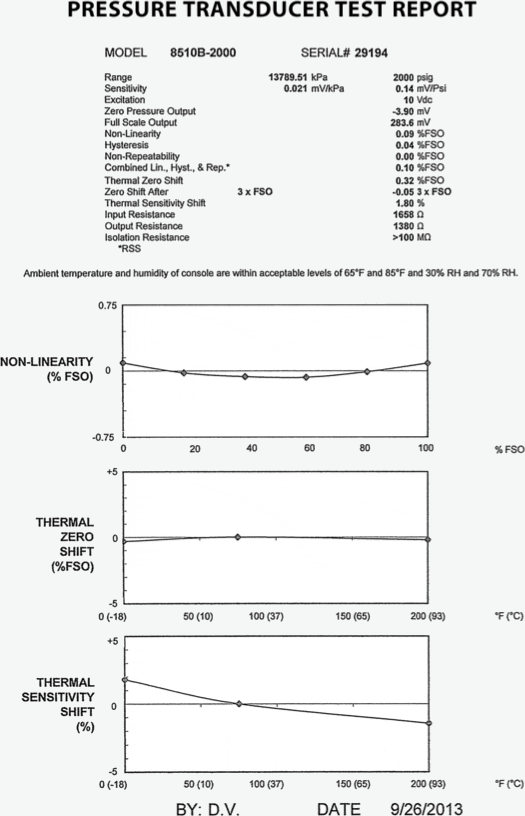Home/Resources/Ask The Experts/Pressure transducer performance explained

# Pressure transducer performance explained

### Question

I am using an 8510B-2000 pressure transducer. Can you explain combined RSS specification? The specification states that I can expect a maximum combined non-linearity, hysteresis and non-repeatability of 0.5%, but by my calculations I get an error of 2.5% (12.5 psi) using 2000 psi as my full scale output.

During the calibration process, Endevco piezoresistive pressure transducers are tested for non-linearity, hysteresis, and non-repeatability. Also provided is the combined uncertainty, which is the root sum squared (RSS) of the above values. The values provided on the product datasheet are typical values. Always use the values supplied on the datasheet since this transducer can have a maximum full-scale voltage of 400 mV and a minimum of 200 mV for 2000 psi. Below is a copy of a typical calibration certificate provided with Endevco pressure transducers:There are two terms that often create confusion:

Full Scale Output (FS0): This is specified in millivolts (mV)

Full Pressure Range: Specified is psi

The above values are calculated from the full-scale output in mV. The model 8510B-2000 has a typical sensitivity of 0.15 mV/g thus the FSO is 0.15mV X 2000 psi which equals 300 mV. For a more accurate number it is recommended that the sensitivity provided on the calibration certificate be used. In this case the typical measurement error is 1% X 300mV = 3 mV.

In the above question, the full scale pressure rating (2000 psi) was used to make the calculation and not the full scale voltage (200 mV).

The user should remember that this error does not take into account the operating temperature. When operating at temperatures other than room temperature; the Endevco calibration certificate provides thermal shift plots to correct for sensitivity and zero shift. The plots cover the compensated temperature range.